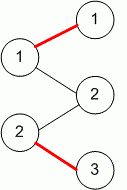Competitions

# Maximum matching

Graph (V, E) is called bipartite, if its set of vertices V can be divided to two subsets A and B so that any edge from E connects vertex from A with vertex from B.

The matching P is any subset E such that no its two edges have common vertex.

Maximum matching is a matching where the number of edges is maximal.

Find the maximal matching in the bipartite graph.

#### Input

The first line contains three numbers n, m и k (1n, m100, 1k10000), where n is a number of vertices in a set A, m is a number of vertices in a set B, and k is a number of edges in a graph. Each of the next k lines contains two numbers ui and vi, meaning that a vertex ui from set A is connected with an edge with vi from set B. The vertices in sets A and B are numbered separately, starting from one. All given numbers are integers.

#### Output

Print in the first line the number of edges l in maximal matching. Then print l rows, two numbers in each. The numbers aj and bj in the j-th row mean that matching includes the edge between vertex aj of the set A and a vertex bj of the set B. Printed edges must form a maximum matching.Time limit 1 second
Memory limit 128 MiB
Input example #1
2 3 4
1 1
1 2
2 2
2 3
Output example #1
2
1 1
2 3